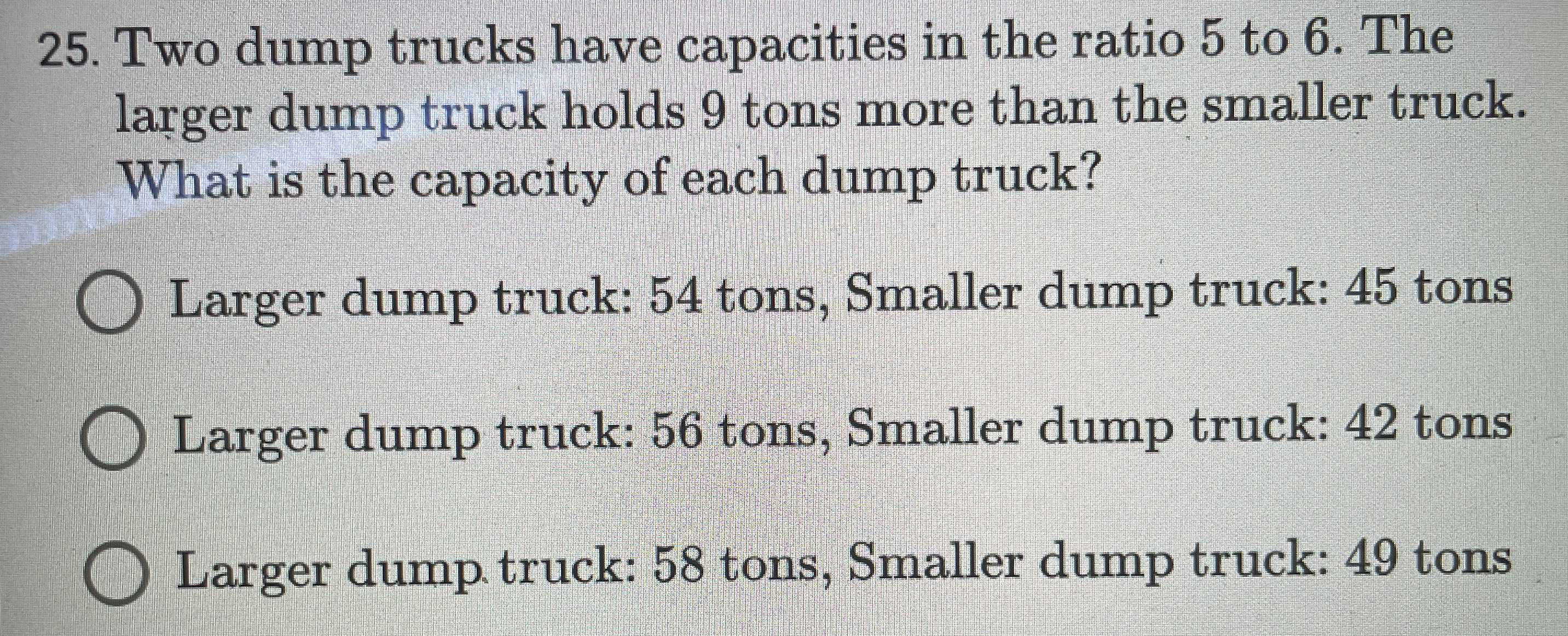### Still have math questions?

Algebra
Question25. Two dump trucks have capacities in the ratio $$5$$ to $$6$$ . The larger dump truck holds $$9$$ tons more than the smaller truck. What is the capacity of each dump truck?

Larger dump truck: $$54$$ tons, Smaller dump truck: $$45$$ tons

Larger dump truck: $$56$$ tons, Smaller dump truck: $$42$$ tons

Larger dump truck: $$58$$ tons, Smaller dump truck: $$49$$ tons

Larger dump truck: $$54$$ tons, Smaller dump truck: $$45$$ tons